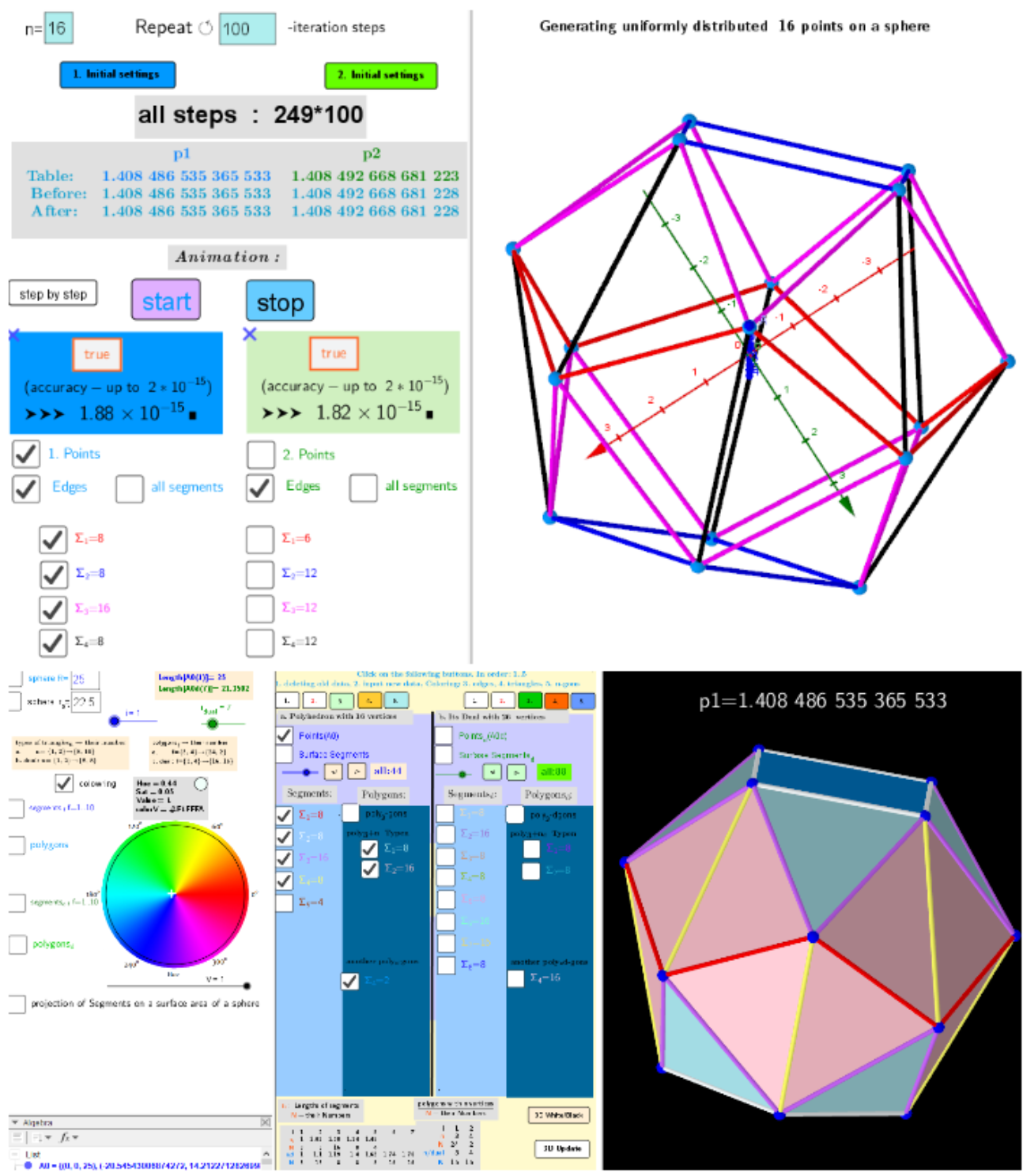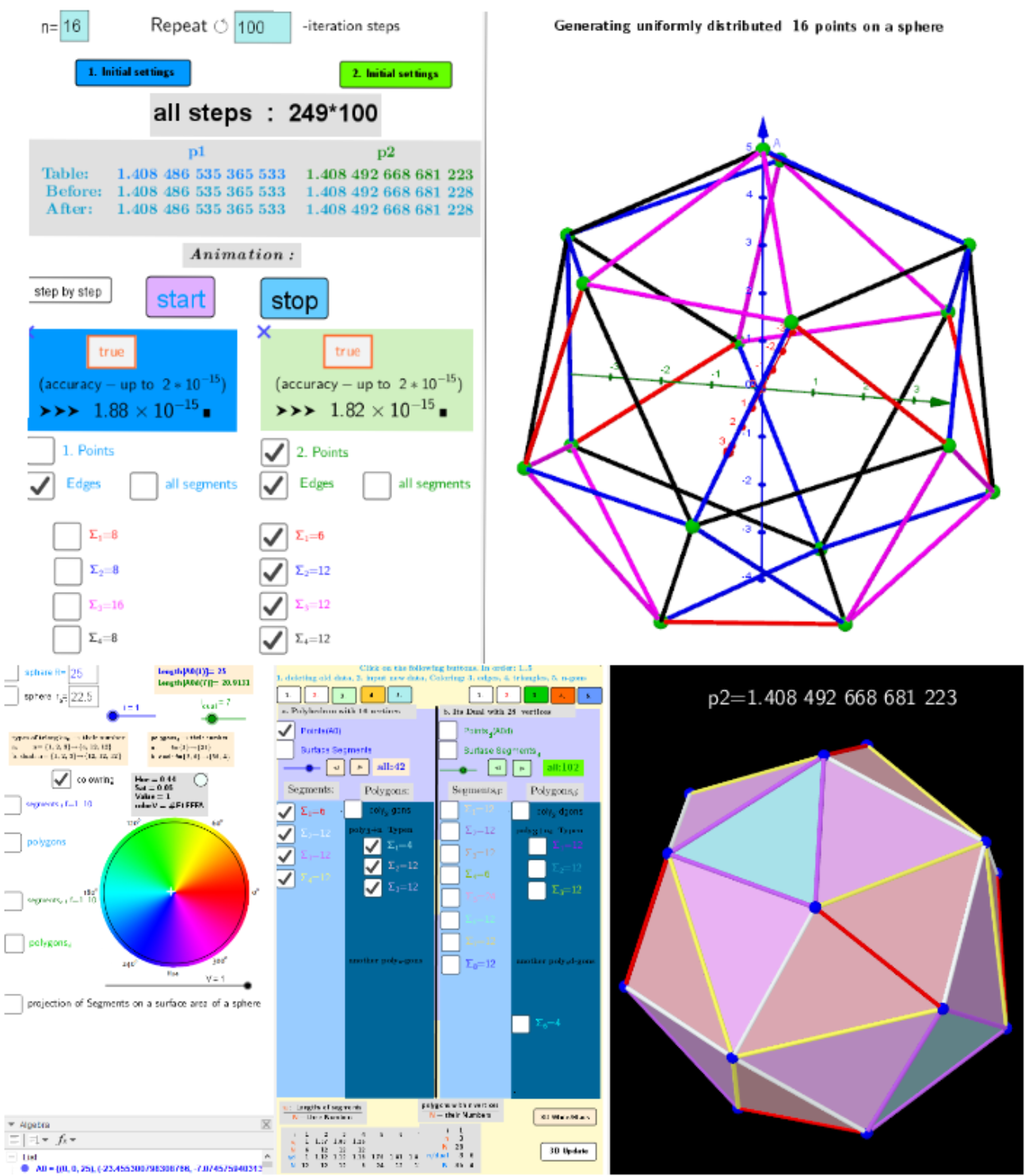# Example of non-uniqueness of the extreme distribution of n=16 particles on the surface of a sphere.

The number of particles on the surface of the sphere is the same. They are placed according to the maximum Distance Sum principle. The "measure" of this distribution is the p-average distance between these particles on the unit sphere. Here, using the example n=16, it is shown, that there are at least two such extreme distributions: p1 = 1.408 486 535 365 533; p2 = 1.408 492 668 681 228. These two distributions are obtained here by a different choice of initial settings-distributions for a further iterative procedure.

## Images: Variant 1p1=1.408 486 535 365 533; Σ1=8, Σ2=8, Σ3=16, Σ4=8 p2=1.408 492 668 681 228; Σ1=6, Σ2=12, Σ3=12, Σ4=12

## Images: Variant 2p1=1.408 486 535 365 533; Σ1=8, Σ2=8, Σ3=16, Σ4=8 p2=1.408 492 668 681 228; Σ1=6, Σ2=12, Σ3=12, Σ4=12